#Pre-Algebra Worksheets

## Linear Functions Worksheets

Here is a graphic preview for all of the Linear Functions Worksheets. You can select different variables to customize these Linear Functions Worksheets for your needs. The Linear Functions Worksheets are randomly created and will never repeat so you have an endless supply of quality Linear Functions Worksheets to use in the classroom or at home.

Our Linear Functions Worksheets are free to download, easy to use, and very flexible.

These Linear Functions Worksheets are a good resource for students in the 5th Grade through the 8th Grade.

Click here for a Detailed Description of all the Linear Functions Worksheets.

## Quick Link for All Linear Functions Worksheets

Click the image to be taken to that Linear Functions Worksheets.

##### Finding  Slope froma Graphed Line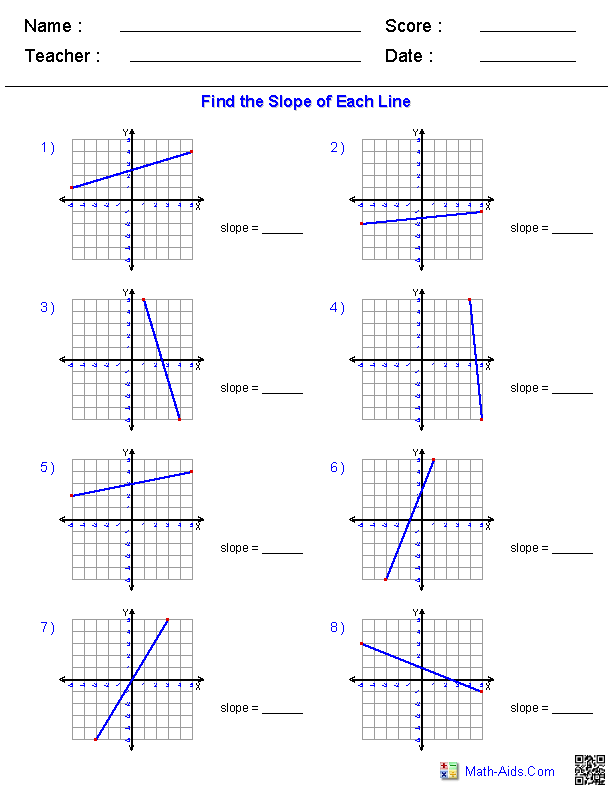##### Finding  Slope froma Pair of Points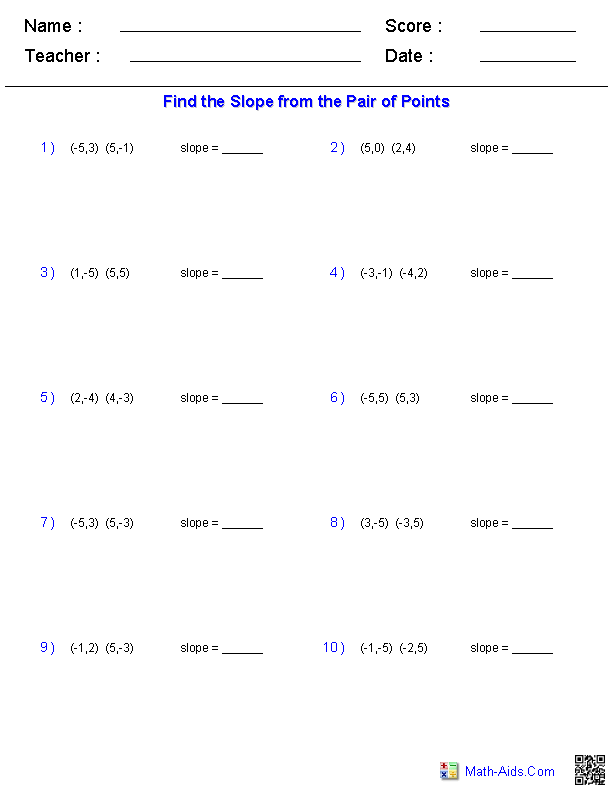##### Finding  Slope and Y-interceptfrom a Linear Equation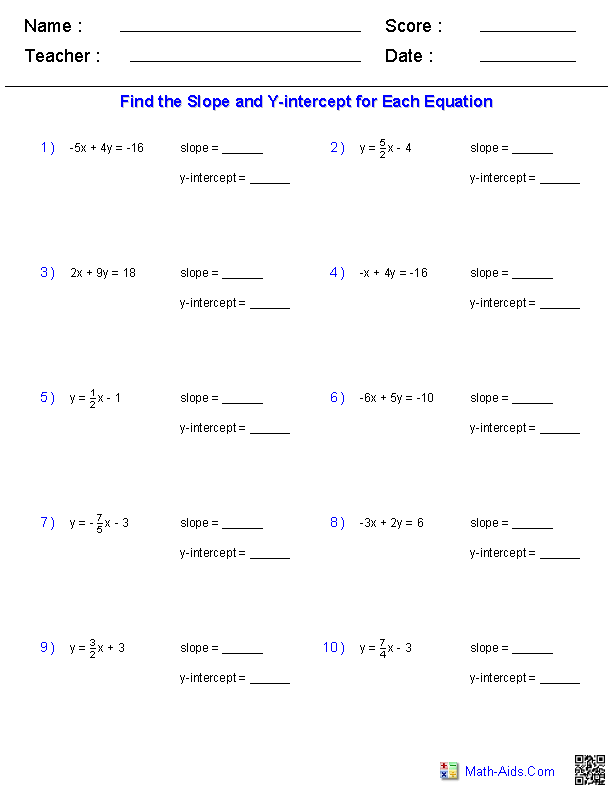##### Graphing  Lines inSlope-Intercept Form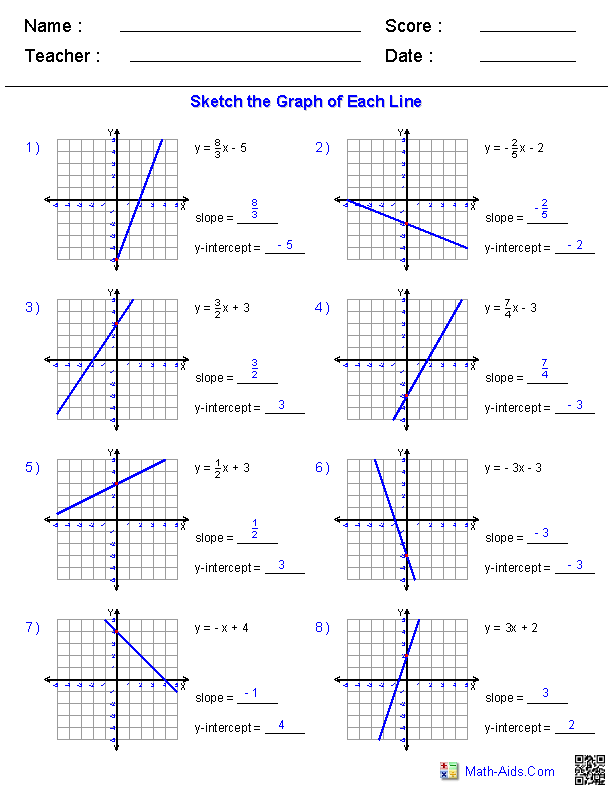##### Graphing  Lines GivenY-Intercept and a Ordered Pair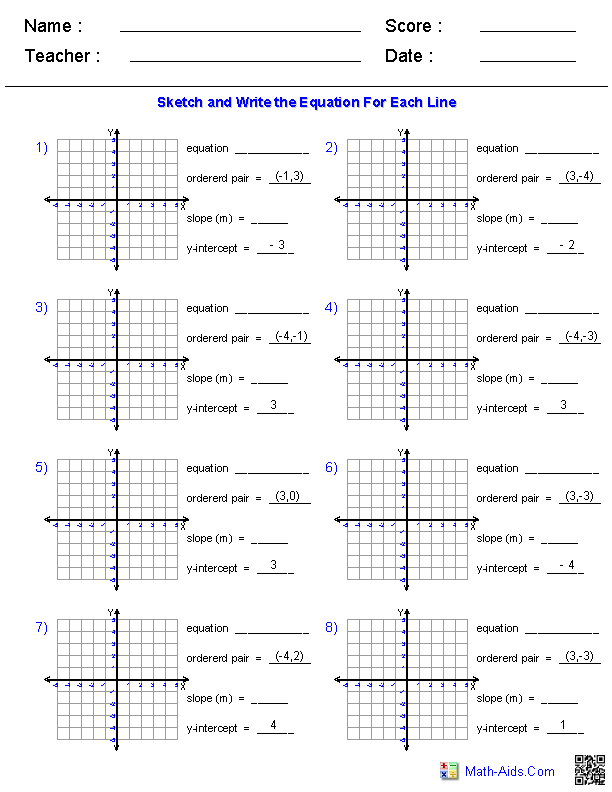##### Graphing  Lines GivenTwo Ordered Pairs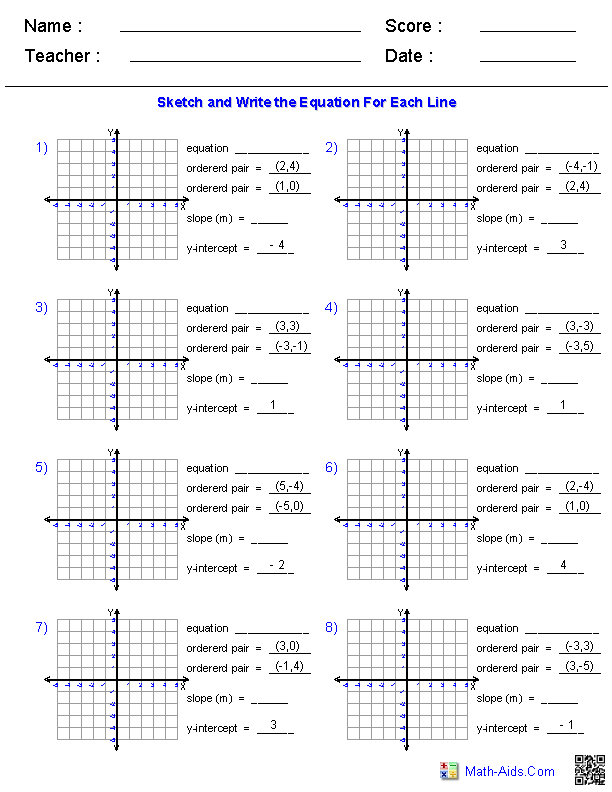##### Graphing  Lines inStandard Form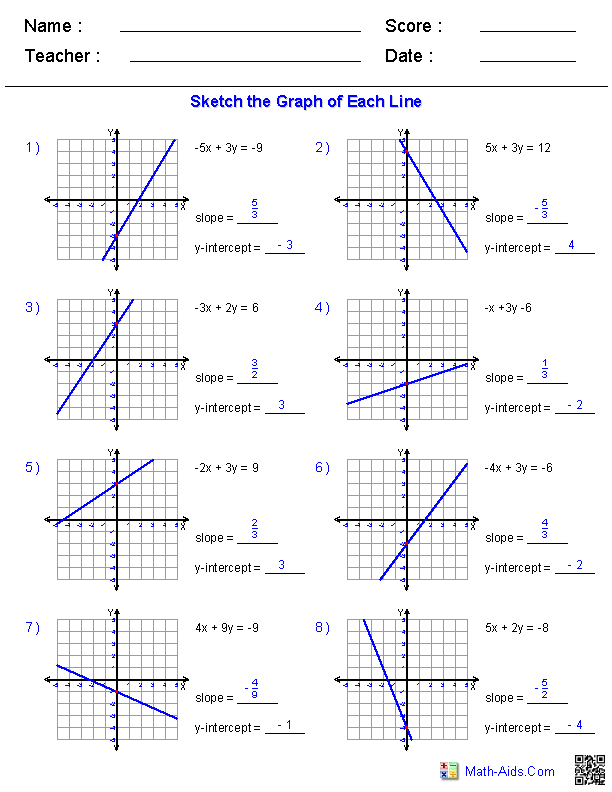##### Working with Linear EquationsLinear Functions Worksheets##### Writing Linear EquationsLinear Functions Worksheets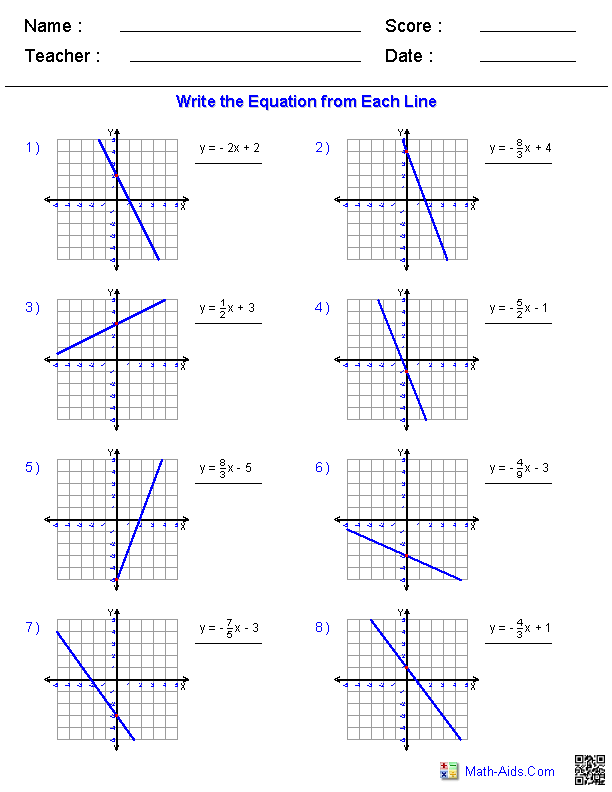##### Graphing Linear InequalitiesLinear Functions Worksheets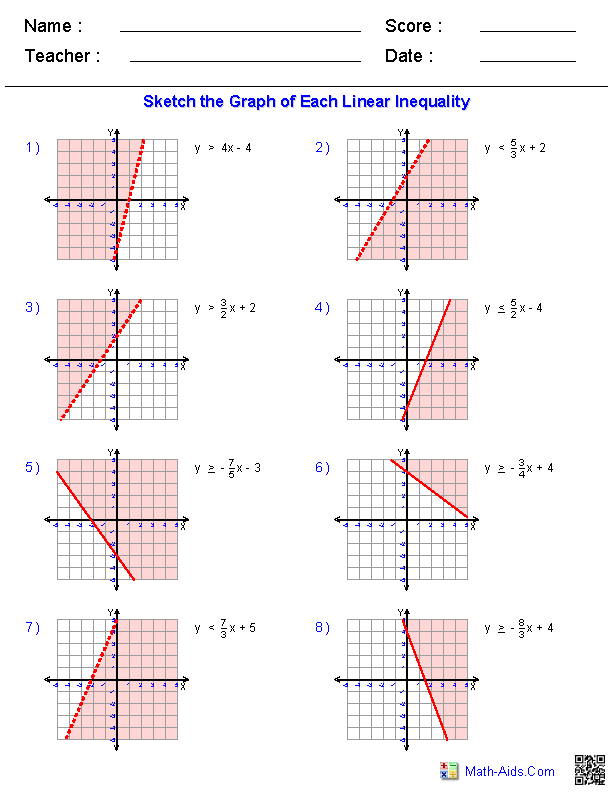Recommended Videos

## Detailed Description for All Linear Functions Worksheets

Finding Slope from a Graphed Line Worksheets
These Linear Functions Worksheets will produce problems for practicing finding the slope from a graphed line. These Linear Functions Worksheets are a good resource for students in the 5th Grade through the 8th Grade.

Finding Slope from a Pair of Points Worksheets
These Linear Functions Worksheets will produce problems for practicing finding the slope from a pair of points. These Linear Functions Worksheets are a good resource for students in the 5th Grade through the 8th Grade.

Finding Slope and the Y-intercept from a Linear Equation Worksheets
These Linear Functions Worksheets will produce problems for practicing finding the slope and Y-intercept from an equation. You may select the type of problems to produce and the solutions that the students must perform. These Linear Functions Worksheets are a good resource for students in the 5th Grade through the 8th Grade.

Graphing Lines in Slope-Intercept Form Worksheets
These Linear Functions Worksheets will produce problems for practicing graphing lines in slope-intercept form. You may select the type of solutions that the students must perform. These Linear Functions Worksheets are a good resource for students in the 5th Grade through the 8th Grade.

Graphing Lines Given Y-intercept and a Ordered Pair Worksheets
These Linear Equations Worksheets will produce problems for practicing graphing lines given the Y-intercept and a ordered pair. You may select the type of solutions that the students must perform. These Linear Equations Worksheets are a good resource for students in the 5th Grade through the 8th Grade.

Graphing Lines Given Two Ordered Pairs Worksheets
These Linear Equations Worksheets will produce problems for practicing graphing lines given two ordered pairs. You may select the type of solutions that the students must perform. These Linear Equations Worksheets are a good resource for students in the 5th Grade through the 8th Grade.

Graphing Lines in Standard Form Worksheets
These Linear Functions Worksheets will produce problems for practicing graphing lines in standard form. You may select the type of solutions that the students must perform. These Linear Functions Worksheets are a good resource for students in the 5th Grade through the 8th Grade.

Working with Linear Equations Worksheets
These Linear Functions Worksheets will produce problems for practicing solving the equation of a linear equation. These Linear Functions Worksheets are a good resource for students in the 5th Grade through the 8th Grade.

Writing Linear Equations Worksheets
These Linear Functions Worksheets will produce problems for practicing writing linear equations from graphed lines. These Linear Functions Worksheets are a good resource for students in the 5th Grade through the 8th Grade.

Graphing Linear Inequalities Worksheets
These Inequality Worksheets will produce problems for practicing graphing linear inequalities. These Inequality Worksheets are a good resource for students in the 5th Grade through the 8th Grade.